# The aspect ratio

The aspect ratio of the rectangular triangle is 13: 12: 5. Calculate the internal angles of the triangle.

Result

A =  90 °
B =  67.38 °
C =  22.62 °

#### Solution:

$a:b:c = 13:12:5 \ \\ a = 13x \ \\ b = 12x \ \\ c = 5x \ \\ \ \\ a^2 = b^2+c^2 \ \\ (13x)^2 = (12x)^2 + (5x)^2 \ \\ 13^2 = 12^2 + 5^2 \ \\ 169 = 144 + 25 \ \\ 169 \ \\ \ \\ A = 90 = 90 ^\circ$
$\sin B = \dfrac{ b }{ a } = \dfrac{ 12x }{ 13x } \ \\ \ \\ B = \dfrac{ 180^\circ }{ \pi } \cdot \arcsin(12/13) \doteq 67.3801 = 67.38 ^\circ = 67^\circ 22'49"$
$C = 90-B = 90-67.3801 = \dfrac{ 1131 }{ 50 } = 22.62 = 22.62 ^\circ = 22^\circ 37'12"$

Try calculation via our triangle calculator.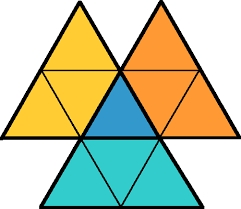Our examples were largely sent or created by pupils and students themselves. Therefore, we would be pleased if you could send us any errors you found, spelling mistakes, or rephasing the example. Thank you!

Leave us a comment of this math problem and its solution (i.e. if it is still somewhat unclear...):Be the first to comment!Tips to related online calculators
Check out our ratio calculator.
Pythagorean theorem is the base for the right triangle calculator.

## Next similar math problems:

1. SSA and geometryThe distance between the points P and Q was 356 m measured in the terrain. The PQ line can be seen from the viewer at a viewing angle of 107° 22 '. The observer's distance from P is 271 m. Determine the viewing angle of P and observer.
2. ObserverThe observer sees straight fence 100 m long in 30° view angle. From one end of the fence is 153 m. How far is it from the another end of the fence?
3. Area and two anglesCalculate the size of all sides and internal angles of a triangle ABC, if it is given by area S = 501.9; and two internal angles α = 15°28' and β = 45°.
4. Inner anglesThe inner angles of the triangle are 30°, 45° and 105° and its longest side is 10 cm. Calculate the length of the shortest side, write the result in cm up to two decimal places.
5. RiverFrom the observatory 11 m high and 24 m from the river bank, river width appears in the visual angle φ = 13°. Calculate width of the river.
6. Largest angle of the triangleCalculate the largest angle of the triangle whose sides have the sizes: 2a, 3/2a, 3a
7. Water channelThe cross section of the water channel is a trapezoid. The width of the bottom is 19.7 m, the water surface width is 28.5 m, the side walls have a slope of 67°30' and 61°15'. Calculate how much water flows through the channel in 5 minutes if the water flow
8. Angles and sides of the triangle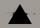Triangle ABC has a circumference of 26 cm. Lengths of the sides are as follows: a = 11.2 cm; b = 6.5 cm. Arrange the interior angles in order of its size. ?
9. Children playgroundThe playground has the shape of a trapezoid, the parallel sides have a length of 36 m and 21 m, the remaining two sides are 14 m long and 16 m long. Determine the size of the inner trapezoid angles.Mast has 13 m long shadow on a slope rising from the mast foot in the direction of the shadow angle at angle 15°. Determine the height of the mast, if the sun above the horizon is at angle 33°. Use the law of sines.The top of the pole we see at an angle of 45°. If we approach the pole by 10 m, we see the top of the pole at an angle of 60°. What is the height of the pole?AC= 40cm , angle DAB=38 , angle DCB=58 , angle DBC=90 , DB is perpendicular on AC , find BD and AD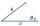The dimensions of the rhomboid sides are a= 5cm, b = 6 cm and the size of the angle at the vertex A is 60°. What is the length of side AC?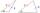Two triangles can be formed with the given information. Use the Law of Sines to solve the triangles. A = 59°, a = 13, b = 14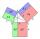From which law follows directly the validity of Pythagoras' theorem in the right triangle? ?Calculate the diamond's diagonal lengths if its content is 156 cm2 and the side length is 13 cm.